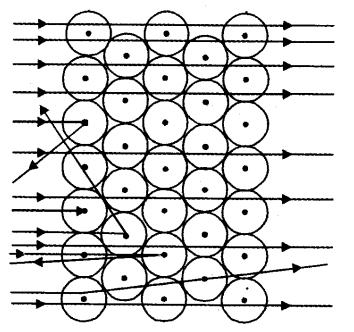# (a) Which popular experiment is shown in the figure ? (b) List three observations of this experiment. (c) State conclusions drawn from each observation of this experiment. (d) State the model of atom suggested on the basis of the above experiment

(a)Which popular experiment is shown in the figure ?
(b) List three observations of this experiment.
© State conclusions drawn from each observation of this experiment.
(d) State the model of atom suggested on the basis of the above experiment.

(a) This figure shows scattering of alfa-particles by a gold foil (Rutherford’s experiment).
(b) Observations:
(i) Most of the a-particles passed straight through the gold foil.
(ii) Some of the a-particles were deflected by the foil by small angles.
(iii) One out of every 12000, particles appeared to rebound.